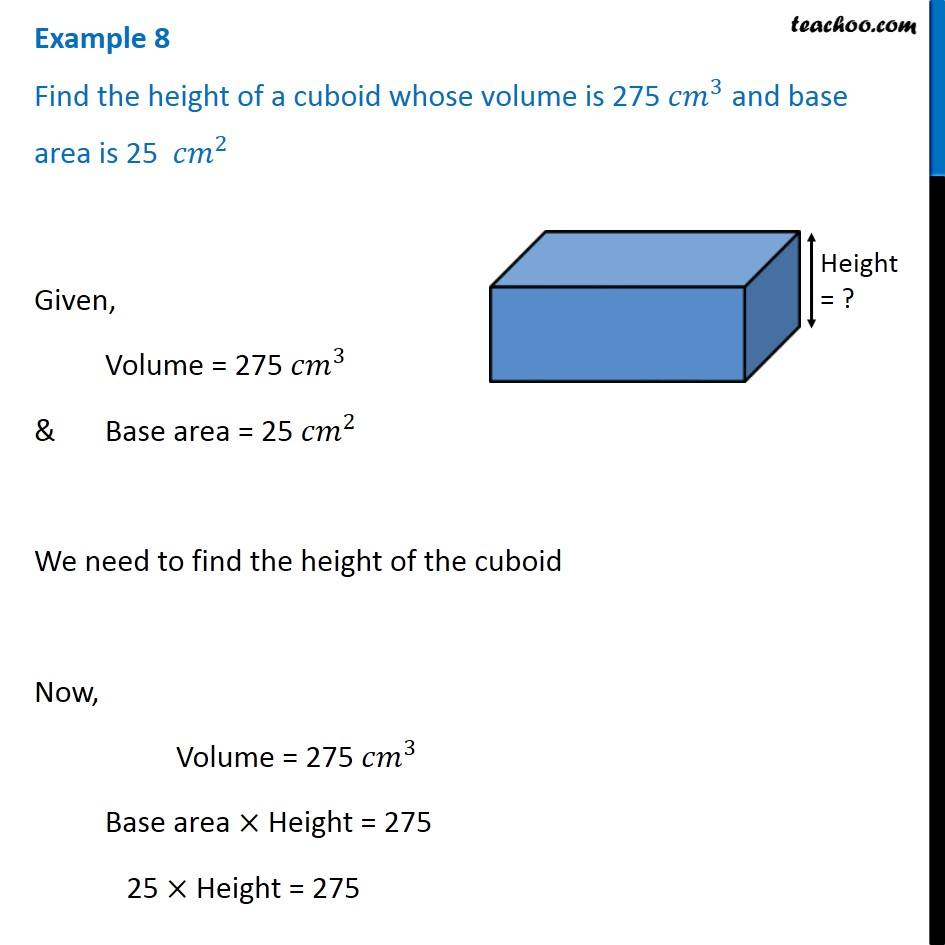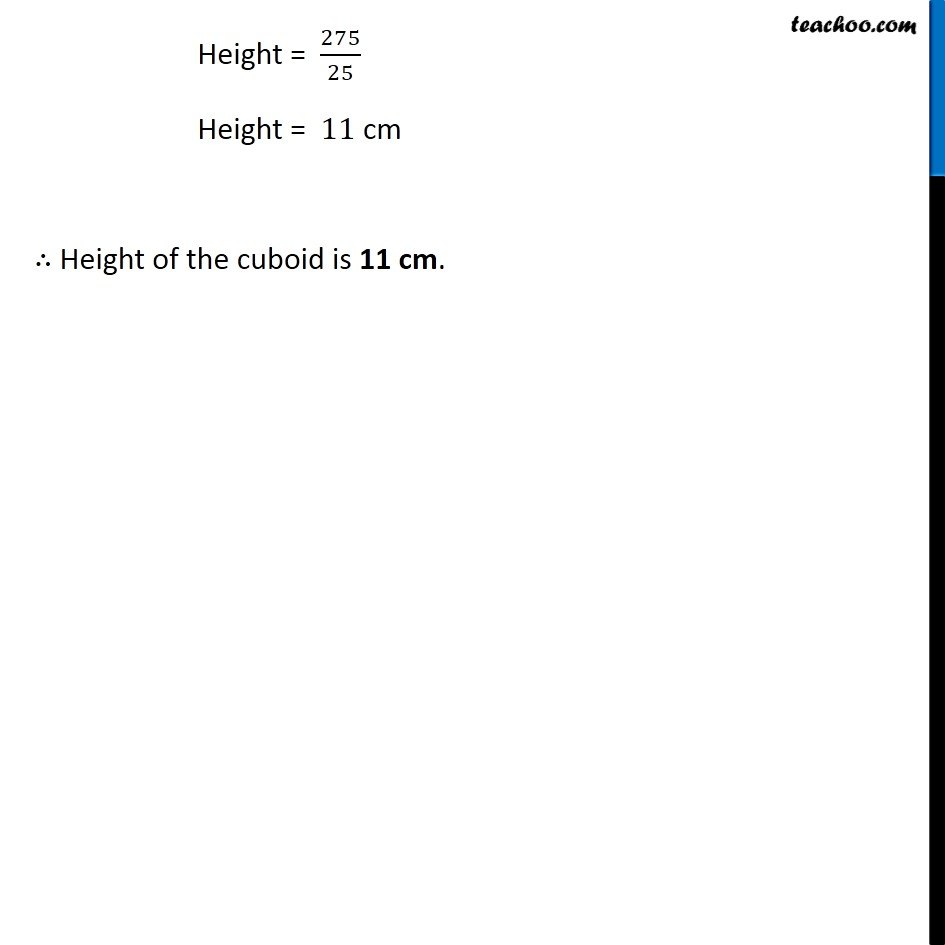Examples

Chapter 11 Class 8 Mensuration
Serial order wise### Transcript

Example 8 Find the height of a cuboid whose volume is 275 〖𝑐𝑚〗^3 and base area is 25 〖𝑐𝑚〗^2 Given, Volume = 275 〖𝑐𝑚〗^3 & Base area = 25 〖𝑐𝑚〗^2 We need to find the height of the cuboid Now, Volume = 275 〖𝑐𝑚〗^3 Base area × Height = 275 25 × Height = 275 Height = 275/25 Height = 11 cm ∴ Height of the cuboid is 11 cm.